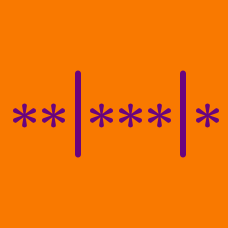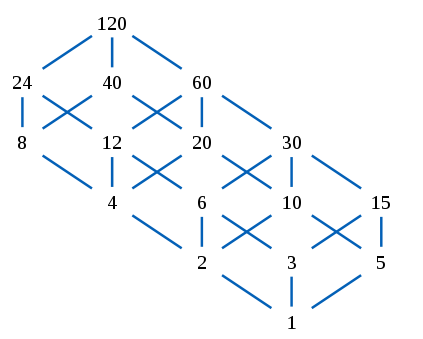Probability

# Distribution into Bins: Level 5 Challenges

$\large A \times B \times C \times D \times E \times F = 7 \times 10^7$

Determine the number of ordered solutions for integers $A,B,C,D,E,F$ such that they satisfy the equation above.

How many 6-digit numbers can be formed using exactly 4 different digits?We want to create a divisible sequence of length $l$ starting from a number $N$. In a Divisible Sequence, every term (except the starting number) is a divisor of the previous term. Examples of divisible sequences of length 3 starting with 10 are:

$10,10,10$

$10,10,5$

$10,10,1$

$10,5,5$ ... etc.

Find the number of divisible sequences of length 5 starting from the number 360.

Seven identical eggs are being put into a $15 \times 1$ egg box. However no more than two eggs can be put in a row. For example, one possible arrangement is E-E----EE-E--EE.

How many possible arrangements are there for the eggs?

Note: The egg box always stays in the same orientation so reflections count.

Let $p(n)$ be the number of partitions of $n$. Let $q(n)$ be the number of partitions of $2n$ into exactly $n$ parts. For example, $q(3) = 3$ because $6 = 4+1+1 = 3+2+1 = 2+2+2.$ Compute $p(12)-q(12).$

Definition: A partition of an integer is an expression of the integer as a sum of one or more positive integers, called parts. Two expressions consisting of the same parts written in a different order are considered the same partition ("order does not matter").

×